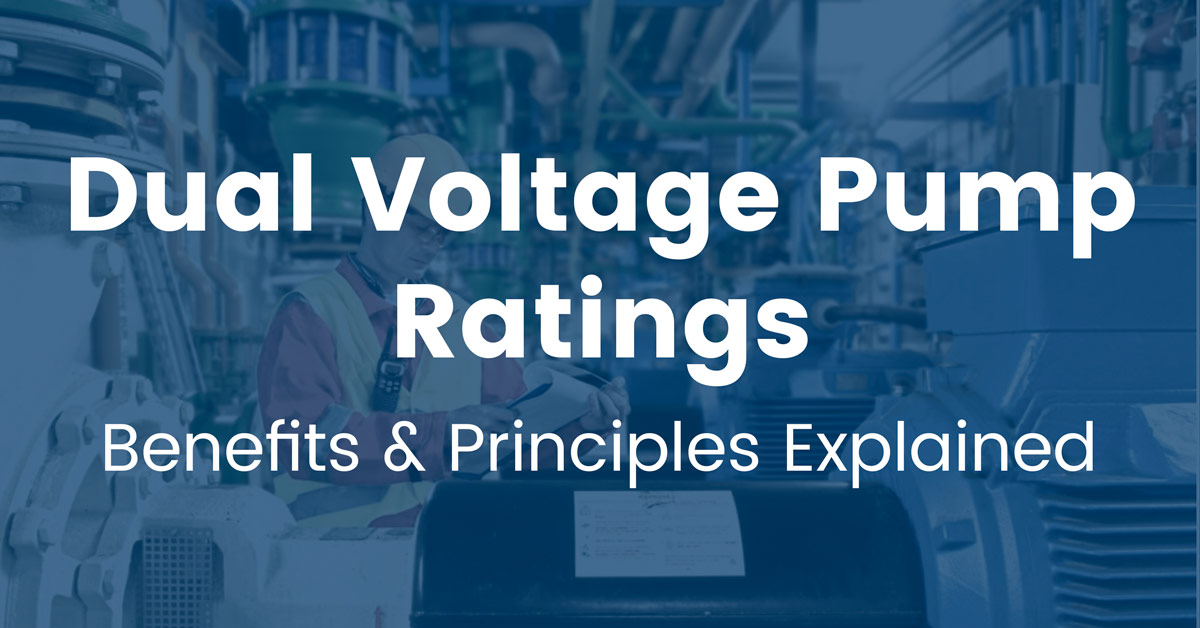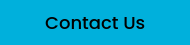# What Dual Voltage Ratings Mean for PumpsChoosing a pump can be confusing if you are unaware of the different pump specifications and categorizations, specifically when it comes to ratings for different voltages (also known as dual voltage pumps).

Can a pump work at two different voltages?

Yes. Let's understand how.

## Understanding Dual Voltage Pumps

When a manufacturer lists something like 208-230/460 on a pump's nameplate, it implies that the pump will run smoothly on either 230 or 460 volts.

If you compare this to a single voltage pump, the advantage is that you have more options when working with a dual voltage pump. However, this feature also affects the pump performance.

## Why Voltage Ratings Matter

In a single voltage pump, manufacturers specify a particular voltage ideal for the pump to function. Most commonly, you'll see these pumps rated for either 115 volts or 230 volts. Although slight variations in these voltages will not affect performance, large voltage fluctuations can.

### Operating Below the Rated Voltage

When the supply voltage in a single voltage pump falls below the rated value, the pump will compensate for the deficiency by drawing more current. A motor will also have a rated amp (current). When the current intake exceeds the rated limit, it will start heating the rotor. With the increase in temperature, the efficiency of the rotor begins deteriorating. With prolonged use, the heat will permanently damage the rotor.

### Operating Above the Rated Voltage

A pump behaves differently when supply voltages are high. You may conclude that with high voltage, the current consumption will decrease. However, that is not the case. With high voltage, the magnets of the motor reach their saturation point. The motor will start drawing large amounts of current at saturation to magnetize the iron and go over the rated limit.

## Dual Voltage Pump Benefits

Now that you know the effects of running a pump beyond its rated voltage limit let's look at dual voltage pumps again.

One of the most significant advantages of dual voltage pumps is that they can run at two different voltages while maintaining the rated output. It doesn't matter if you run the pump at the higher or lower voltage limit; the power pump receives the same amount of power. A dual voltage pump achieves this by compensating voltage for power.

## How Does a Dual Voltage Pump Balance between Power and Voltage?

To understand the relationship between voltage and power in the context of a pump, let's look at the equation for power:

P (Power) = V (Voltage) x I (Current)

From the equation, you can see that power generated by a pump is the product of voltage and current. If the value of P goes down, then the value of I must increase to produce the same amount of power.

To simplify further, consider the power needed from the pump as 60 units. The pump now operates with 20 units of voltage and 3 units of current. The equation is balanced in this case.

However, if the voltage goes down to 10 units, the pump must increase its current intake to 6 units to achieve the same power. This also results in a balance.

## How Can a Pump Operate at Dual Voltage?

As discussed already, not all pumps can work at dual voltages. And the reason for this exclusivity boils down to the type of winding that a motor has. These windings are connected either in parallel for the low voltage (110V) or in series for the high voltage (230V). Hence, the pump has a fixed voltage rating.

Three-phase motors can have Y, Delta, or Y/Delta winding connections, maintaining a 1:2 ratio. That is why you'll see most pumps with three-phased motors rated for 208-230/460 Volts.